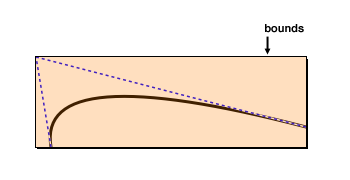### java.awt.geom

• `java.lang.Object`
• `java.awt.geom.QuadCurve2D`
• `java.awt.geom.QuadCurve2D.Float`
Enclosing Class:
Implemented Interfaces:
Cloneable, Shape

`public static class QuadCurve2D.Float`
`extends QuadCurve2D`

A two-dimensional curve that is parameterized with a quadratic function and stores coordinate values in single-precision floating-point format.
`QuadCurve2D.Double`

## Nested Class Summary

### Nested classes/interfaces inherited from class java.awt.geom.QuadCurve2D

`QuadCurve2D.Double`, `QuadCurve2D.Float`

## Field Summary

` float`
`ctrlx`
The x coordinate of the curve’s control point.
` float`
`ctrly`
The y coordinate of the curve’s control point.
` float`
`x1`
The x coordinate of the curve’s start point.
` float`
`x2`
The x coordinate of the curve’s end point.
` float`
`y1`
The y coordinate of the curve’s start point.
` float`
`y2`
The y coordinate of the curve’s end point.

## Constructor Summary

`Float()`
Constructs a new QuadCurve2D that stores its coordinate values in single-precision floating-point format.
`Float(float x1, float y1, float cx, float cy, float x2, float y2)`
Constructs a new QuadCurve2D that stores its coordinate values in single-precision floating-point format, specifying the initial position of each point.

## Method Summary

` Rectangle2D`
`getBounds2D()`
Determines the smallest rectangle that encloses the curve’s start, end and control point.
` Point2D`
`getCtrlPt()`
Returns the curve’s control point.
` double`
`getCtrlX()`
Returns the x coordinate of the curve’s control point.
` double`
`getCtrlY()`
Returns the y coordinate of the curve’s control point.
` Point2D`
`getP1()`
Returns the curve’s start point.
` Point2D`
`getP2()`
Returns the curve’s end point.
` double`
`getX1()`
Returns the x coordinate of the curve’s start point.
` double`
`getX2()`
Returns the x coordinate of the curve’s end point.
` double`
`getY1()`
Returns the y coordinate of the curve’s start point.
` double`
`getY2()`
Returns the y coordinate of the curve’s end point.
` void`
`setCurve(double x1, double y1, double cx, double cy, double x2, double y2)`
Changes the geometry of the curve, specifying coordinate values as double-precision floating-point numbers.
` void`
`setCurve(float x1, float y1, float cx, float cy, float x2, float y2)`
Changes the geometry of the curve, specifying coordinate values as single-precision floating-point numbers.

### Methods inherited from class java.awt.geom.QuadCurve2D

`clone`, `contains`, `contains`, `contains`, `contains`, `getBounds`, `getCtrlPt`, `getCtrlX`, `getCtrlY`, `getFlatness`, `getFlatness`, `getFlatness`, `getFlatnessSq`, `getFlatnessSq`, `getFlatnessSq`, `getP1`, `getP2`, `getPathIterator`, `getPathIterator`, `getX1`, `getX2`, `getY1`, `getY2`, `intersects`, `intersects`, `setCurve`, `setCurve`, `setCurve`, `setCurve`, `setCurve`, `solveQuadratic`, `solveQuadratic`, `subdivide`, `subdivide`, `subdivide`

### Methods inherited from class java.lang.Object

`clone`, `equals`, `extends Object> getClass`, `finalize`, `hashCode`, `notify`, `notifyAll`, `toString`, `wait`, `wait`, `wait`

## Field Details

### ctrlx

`public float ctrlx`
The x coordinate of the curve’s control point.

### ctrly

`public float ctrly`
The y coordinate of the curve’s control point.

### x1

`public float x1`
The x coordinate of the curve’s start point.

### x2

`public float x2`
The x coordinate of the curve’s end point.

### y1

`public float y1`
The y coordinate of the curve’s start point.

### y2

`public float y2`
The y coordinate of the curve’s end point.

## Constructor Details

### Float

`public Float()`
Constructs a new QuadCurve2D that stores its coordinate values in single-precision floating-point format. All points are initially at position (0, 0).

### Float

```public Float(float x1,
float y1,
float cx,
float cy,
float x2,
float y2)```
Constructs a new QuadCurve2D that stores its coordinate values in single-precision floating-point format, specifying the initial position of each point.
Parameters:
`x1` - the x coordinate of the curve’s start point.
`y1` - the y coordinate of the curve’s start point.
`cx` - the x coordinate of the curve’s control point.
`cy` - the y coordinate of the curve’s control point.
`x2` - the x coordinate of the curve’s end point.
`y2` - the y coordinate of the curve’s end point.

## Method Details

### getBounds2D

`public Rectangle2D getBounds2D()`
Determines the smallest rectangle that encloses the curve’s start, end and control point. As the illustration below shows, the invisible control point may cause the bounds to be much larger than the area that is actually covered by the curve.Specified by:
getBounds2D in interface Shape

### getCtrlPt

`public Point2D getCtrlPt()`
Returns the curve’s control point.
Overrides:

### getCtrlX

`public double getCtrlX()`
Returns the x coordinate of the curve’s control point.
Overrides:

### getCtrlY

`public double getCtrlY()`
Returns the y coordinate of the curve’s control point.
Overrides:

### getP1

`public Point2D getP1()`
Returns the curve’s start point.
Overrides:

### getP2

`public Point2D getP2()`
Returns the curve’s end point.
Overrides:

### getX1

`public double getX1()`
Returns the x coordinate of the curve’s start point.
Overrides:

### getX2

`public double getX2()`
Returns the x coordinate of the curve’s end point.
Overrides:

### getY1

`public double getY1()`
Returns the y coordinate of the curve’s start point.
Overrides:

### getY2

`public double getY2()`
Returns the y coordinate of the curve’s end point.
Overrides:

### setCurve

```public void setCurve(double x1,
double y1,
double cx,
double cy,
double x2,
double y2)```
Changes the geometry of the curve, specifying coordinate values as double-precision floating-point numbers.
Overrides:
Parameters:
`x1` - the x coordinate of the curve’s new start point.
`y1` - the y coordinate of the curve’s new start point.
`cx` - the x coordinate of the curve’s new control point.
`cy` - the y coordinate of the curve’s new control point.
`x2` - the x coordinate of the curve’s new end point.
`y2` - the y coordinate of the curve’s new end point.

### setCurve

```public void setCurve(float x1,
float y1,
float cx,
float cy,
float x2,
float y2)```
Changes the geometry of the curve, specifying coordinate values as single-precision floating-point numbers.
Parameters:
`x1` - the x coordinate of the curve’s new start point.
`y1` - the y coordinate of the curve’s new start point.
`cx` - the x coordinate of the curve’s new control point.
`cy` - the y coordinate of the curve’s new control point.
`x2` - the x coordinate of the curve’s new end point.
`y2` - the y coordinate of the curve’s new end point.